[BACK]
 Computers, Materials & ContinuaDOI:10.32604/cmc.2022.019884Article

DLBT: Deep Learning-Based Transformer to Generate Pseudo-Code from Source Code

1Faculty of Computers and Information Sciences, Ain Shams University, Abassia, Cairo, 11566, Egypt
2College of Computer Engineering and Sciences, Prince Sattam Bin Abdulaziz University, Al Kharj, 11942, Saudi Arabia
Received: 29 April 2021; Accepted: 21 June 2021

Abstract: Understanding the content of the source code and its regular expression is very difficult when they are written in an unfamiliar language. Pseudo-code explains and describes the content of the code without using syntax or programming language technologies. However, writing Pseudo-code to each code instruction is laborious. Recently, neural machine translation is used to generate textual descriptions for the source code. In this paper, a novel deep learning-based transformer (DLBT) model is proposed for automatic Pseudo-code generation from the source code. The proposed model uses deep learning which is based on Neural Machine Translation (NMT) to work as a language translator. The DLBT is based on the transformer which is an encoder-decoder structure. There are three major components: tokenizer and embeddings, transformer, and post-processing. Each code line is tokenized to dense vector. Then transformer captures the relatedness between the source code and the matching Pseudo-code without the need of Recurrent Neural Network (RNN). At the post-processing step, the generated Pseudo-code is optimized. The proposed model is assessed using a real Python dataset, which contains more than 18,800 lines of a source code written in Python. The experiments show promising performance results compared with other machine translation methods such as Recurrent Neural Network (RNN). The proposed DLBT records 47.32, 68. 49 accuracy and BLEU performance measures, respectively.

Keywords: Natural language processing; long short-term memory; neural machine translation; pseudo-code generation; deep learning-based transformer

1  Introduction

In the software development cycle , there are many different ways of writing code based on the syntax of the programming language. Understanding the program is challenging if the developer is unfamiliar with the programming language. Software development is a collaborative process involving many developers. At any time, the code is changed according to the requirements updates. Before any change, the developer would need to read all the previous lines of code to make the new updates. Therefore, developers should associate a description with each code line they write, which makes code updating is very difficult. This process is difficult and ignored by majority of programmers. Thus, many tools and techniques are developed to automatically generate textual descriptions of the source code. This process is done at the level of accurate code statements [2,3]. Pseudo-code is a well-known textual description for source code. This description uses natural language and mathematical expressions to explain what the source code does. Moreover, Pseudo-code can be converted into any programming language as shown in Fig. 1. This an example of the source Python code and its equivalent in Pseudo-code. Python code instruction “self . _servers = server . split ( ′;″ )” is written in Pseudo-code “split server with ′;″ as a delimiter, substitute the result for self._servers.”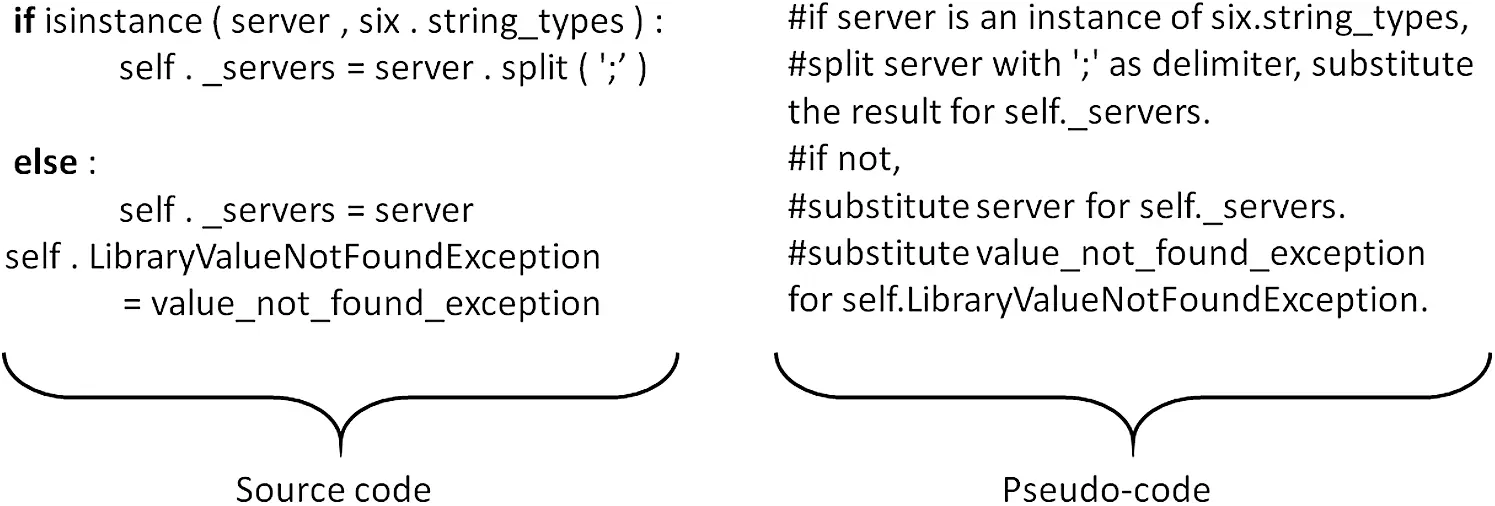Figure 1: An example of Python code and its corresponding Pseudo-code

Therefore, an automatic way to generating Pseudo-code from the source code is needed. Furthermore, to generate the Pseudo-code from the source code, Statistical Machine Translation (SMT) is used , which is a process of machine translation. Another way to solve this problem is the rule-based machine, which is statistically drawn from the source and target sentences and creates its own translation. Deep learning  is used in machine translation, known by the name of Neural Machine Translation (NMT). This is the current alternative to Statistical Machine Translation (SMT) because it gives better results . The most popular model in the NMT is Recurrent Neural Network (RNN)  in which the translation data follows a sequential and temporary pattern. The problem in the RNN is the training process, as weights tuning has a very small value which is called vanishing gradient , or weight changes to a large value which is called exploding gradient. To solve this problem, the Long Short-Term Memory (LSTM) model is used  as it uses language modeling . This leads to the success of backpropagation to optimize the weights . The major limitations of LSTM-based models are that it processes the code sequentially and fails to capture the long-term dependencies between code tokens.

In this paper, a novel deep learning-based transformer (DLBT) model is proposed for automatic Pseudo-code generation from the source code. The proposed model is a transformer machine translation model that is based on deep learning  to overcome the limits of the RNN and LSTM models. The DLBT model is built on the sequence-to-sequence frame and is based on the attention layer without the need for a Recurrent Neural Network (RNN). The proposed DLBT is an encoder-decoder structure, which is consists of three components: Tokenization and Embedding, Transformer (encoding and decoding) and Post-processing. Each sentence is separated in tokens, and positional encoding is applied to prepare the input entry for the transformer step. Then, the encoder processes the input iteratively, then the decoder does the same at the output of the encoder. Finally, a correction function is applied to enhance the resultant Pseudo-code. The proposed model is evaluated using a large dataset of Python code . The BLEU  is used to measure the Pseudo-code quality. The results of experiments are promising compared to other Neural Machine Translation (NMT) methods. The proposed DLBT records 47.32, 68. 49 accuracy and BLEU performance measures, respectively.

The main goal of the proposed model is to automatically generate the Pseudo-code by avoiding the problem of the vanishing gradient because it has access at each layer of all input tokens. In addition, the DLBT uses the attention layer independently of the previous state. This independence leads to lower computation and speed in training by avoiding weight calculations between the situations or using a long short-term memory.

This paper is organized as follows. Section 2 presents a literature review. Section 3 presents the DLBT proposed model. Section 4 shows experimental results, and finally, Section 5 is the conclusions.

2  Related Work

Machine Translation (MT) is used to translate a language into other languages. The methods which are based on Machine Translation are comprised of rule-based and SMT. Recently the Neural Machine Translation (NMT), has increased for language modeling. In , authors generated Pseudo-code from the Python source code using NMT. Their approach is based on the LSTM model and the attention layer. LSTM is used to learn long-term dependencies in the source code. Many code elements are dependent although they are in different order. The attention layer is applied to the encoder-decoder model as it improves the performance of the translation . It is also used to align incoming and outcoming sequences. In , the Pseudo-code is generated from the Python source code with Statistical Machine Translation (SMT). This method involves Phrase-Based Machine Translation and Tree-to String Machine Translation. Phrase-Based Machine Translation relates the phrases of the input source code with the phrases of the target of the corresponding Pseudo-code. In addition, Tree-to String Machine Translation converts the code into tree using Abstract Syntax Trees (AST)  to maintain the structural context of the code. Tree-to String Machine Translation has three different styles: raw code trees, inserted tree heads, and reduced trees.

In , the authors designed a model for generating Pseudo-code that consists of three parts: an input encoder, a sketch decoder, and a natural language explanation decoder. The input encoder is used to encode the embedding vector of all the input data in a latent vector space. Then, bidirectional LSTM (BiLSTM) is used to encode the input embedding. The sketch decoder adds LSTM with an attention mechanism. The natural language explanation decoder is based on recurrent neural networks (RNN), an attention mechanism, and a copy mechanism . In , authors generated Python source code from Pseudo-code using NMT. Their approach is made of two frameworks: The grammatical model and the deep learning model. The grammar model is applied in source code sentences after conversion into a tree by ASTs. String-to-Tree Machine Translation has two actions that run in the grammar model APPLYULE [r] and GENTOKEN [v]. APPLYULE [r] extracts the role of the structure of the code in current ASTs. The result of this action will be the roles, call function, if-statement, loop, or function definition. GENTOKEN [v] defines the value of a node within AST from a token word. Neural Machine Translation (NMT) works in String-to-Tree Machine Translation in the encoder and decoder. Encoder reserves the input as a sequence of the words and uses bidirectional LSTM. The decoder presents the RNN model at AST using the vertical LSTM and applies the Grammar model for each node in AST. The connection between encoder and decoder is done using Deep Neural Network (DNN).

The authors in , started with the syntactic code generation model in  which uses sequences of actions to generate the AST before converting it to a code. Then, the Retrieval-Based mechanism  is applied to improve the result when the input is similar to the input in the training dataset but does not exist as it applies a tree instead of a string. The Retrieval-Based code generation RECODE has four steps to achieve. First, retrieve the most similar Pseudo-code from the training Pseudo-code set compared to the input sentence. Second, translate the retrieved sentences from the first step and have the ASTs to extract n-gram of a subtree. Third, words that are similar between the output and the retrieved sentence are searched for by input and then replaced by words that are not the same between the input sentence and the retrieved sentence in the first step. Fourth, each decoding step increases the probability of the action if the same procedure is repeated to call a later time.

The work of  introduced a Tree-Based Convolutional Neural Network (TBCNN) for classifying programs based on code functionality and program detection. This could be done by converting the code to a tree by using ASTs and representing the nodes to vector. This shape of vectors such as a triangle as tree-based convolution, is applied by max-pooling. Dynamic pooling is the result of the previous step, then it adds a fully-connected hidden layer and a SoftMax as the output layer in the architecture. The work in  presents a method for generating code comments for Java methods through the introduced model which is called DeepCom. The framework of DeepCom has three steps: data processing, training a sequence-to-sequence model, and generating comments with the trained model. In the data processing step, the data of the source files must be obtained and separated into natural language annotation and java code. The java method converts AST data into a string and is inputted in the training a sequence-to-sequence model as x. The natural language annotation is inputted in the training a sequence-to-sequence model as y. Training a sequence-to-sequence model is followed by the NMT architecture and uses LSTM in each encoder and decoder. After training the model and inputting a java method into the model, the comment is generated. In , DeepCom is developed to represent the code structure as a tree using AST. In , the DeepCom is enhanced for Hybrid-DeepCom. It consists of three components: local encoder that is based on a bi-directional Gated Recurrent Unit (bi-GRU) , global encoder which is built upon a Graph Attention (GAT) network , and decoder that aggregates the local and global information learned by the local and global encoders. The work in  presents a rule-based method for generating Pseudo-code from the Python source code. The dataset which is used in this work is the same dataset in [2,3]. Tab. 1 shows a summary comparison between different machine translation methods.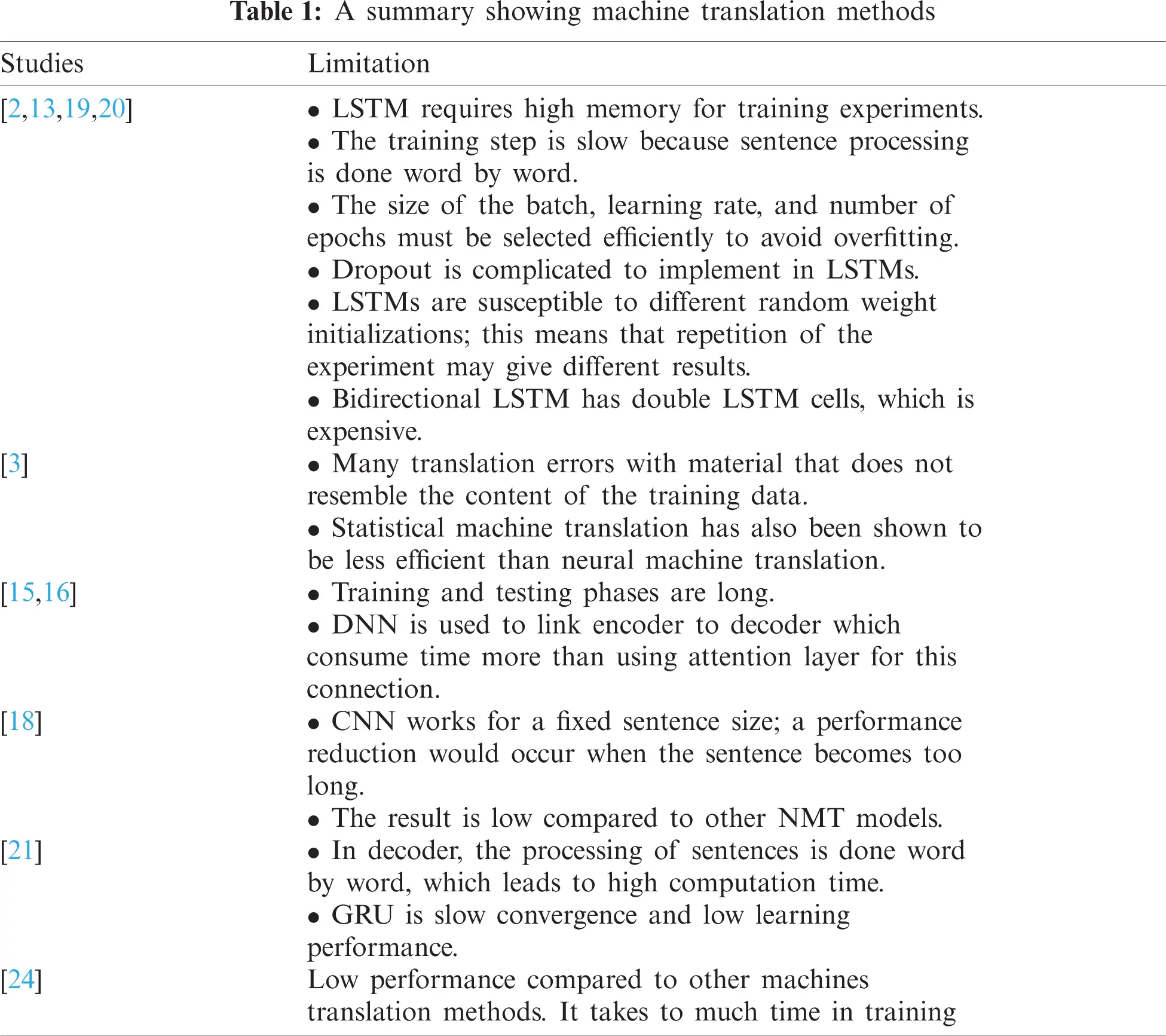3  Deep Learning-Based Transformer (DLBT) Model

The proposed Deep Learning-Based Transformer (DLBT) model generates Pseudo-code from the source code based on the Transformer Neural Machine Translation (TNMT). The proposed model is organized as a stack of self-attention and pointwise, fully connected layers as shown in Fig. 2. The DLBT consists of the following components:

●   Tokenization and Embedding.

●   Transformer (encoding and decoding).

●   Post-processing.

3.1 Tokenization and Embedding

In this step, each sentence is tokenized. For example, the sentence s = s.replace ( ′ \t″ , ′\ \t″ ) is tokenized as [′s″,'=″,'s″,″.″,'replace″,″′,'(′, ′\'″,'\″,'t″,'\'″,″,″,'\'″,'\″,'\″,'t″,'\'″,″)″]. The order of the tokens are unimportant, because the input is represented as a set of tokens. Two different methods are used to tokenize:

●   Method 1, the rule expression of the Tokenizer is ([∧A-Za-z0-9_:.]);

●   Method 2, the rule expression of Tokenizer is (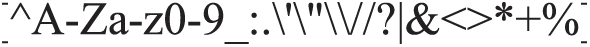).

For example, if there are three statements: “ the_expression=(′?\/∗+−%<>:&|″) ”, “ default: “html,txt”, or “js” ”, and “z2=x1+y3”. The output of the tokenization step after applying Method 1 will be as follows:Figure 2: The proposed deep learning-based transformer (DLBT) model architecture

●   Statement 1: [the_expression, =, (, ′, ?, V, ∗, +, -, %, <, >, :, &, |, ′, )];

●   Statement 2: [default:, “, html, ′,″, txt, “, ′,″, or, “, js, “];

●   Statement3: [z2, =, x1, +, y3].

The result of the tokenizing step after applying Method 2 will be the following:

●   Statement 1:[the_expression, =, (, ′?/,⋁∗+-%<>:&|″,)];

●   Statement 2: [default:, “html, ′,″, ′txt∁, ′,″, ′or″, “js∁];

●   Statement 3: [z2, =, x1+y3].

Both methods are used for Pseudo-code and code. Finally, additional tokens are added at the beginning and end of the sequence. For example, <sop>for start-of-Pseudo-code and <eop>for end-of-Pseudo-code. After tokenization, tokens embedding is applied. This is a way to represent similar tokens by similar encodings. A floating-point value is assigned to similar tokens constructing a dense vector. Finally, positional encoding is done to discover the sequence order by alternating the embeddings depending on the position. Before the first attention layer, a small set of constants is added to the embedding vector as in , the sinusoidal function is used for the positional encoding.

For example, the sentence “I love the Transformer model “is tokenized as [I, love, the, Transformer, model]. Then, each token is assigned an index such as indexes in the list [224,378,962,1123,4136]. Each token is initialized a d-length vector, which has a random number from −1 to 1. Each vector value represents the linguistic features that are used during the training step. If two tokens share similar language characteristics and appear in similar contexts, their embedding values are updated to be closer during the training process. Fig. 3 shows the embedding process assuming d = 5.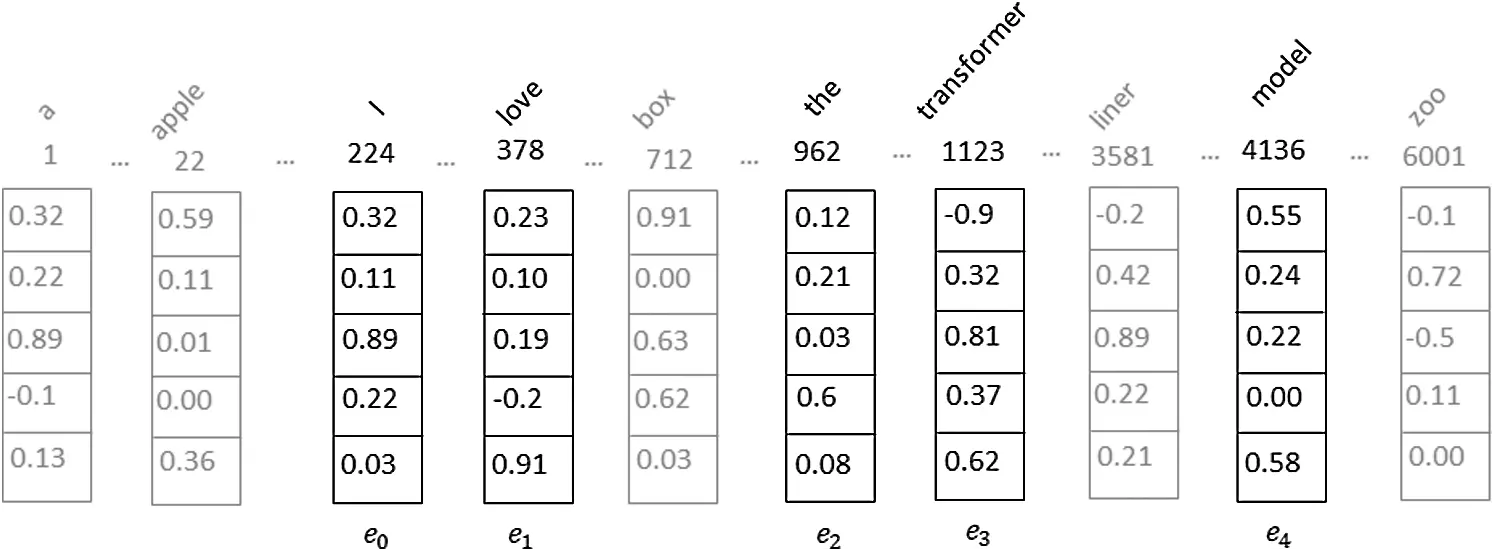Figure 3: Embedding process for “I love the transformer model” sentence

In positional encodings, Eqs. (1) and (2) are used as follows:

PE(pos,2i)=sin(pos100002id)(1)

PE(pos,2i+1)=cos(pos100002id)(2)

where pos is the position word in the sentence, i is index in vector of position, and d is length of position vector. In , the authors hypothesized the Eq. (1) used for the odd index, the Eq. (2) for even index and d value = 512 would make the model easy to learn. Fig. 4 shows positional encodings steps to sentence “I love transformer the model” how will be with addition position.

3.2 Transformer (Encoding & Decoding)

The DLBT is an encoder-decoder frame structure. Encoder processes the input iteratively, then the decoder does the same to the output of the encoder. Encoder and decoder are composed of uniformly chained layers. The encoder and decoder use attention mechanism to weigh each input, the relevance of each other input, and pull information from them accordingly to produce the result. In addition, the feed-forward neural network is used in both the encoder and decoder to perform the additional processing of outputs. Residual connection and normalization layer  are used for connecting sub-layers. The encoder function is to process input vectors and generates information about inputs parts that are relevant to each other, then the generated information is the input for the next encoder. On the other hand, decoders do the opposite. Each encoder takes the generated information and generates an output sequence.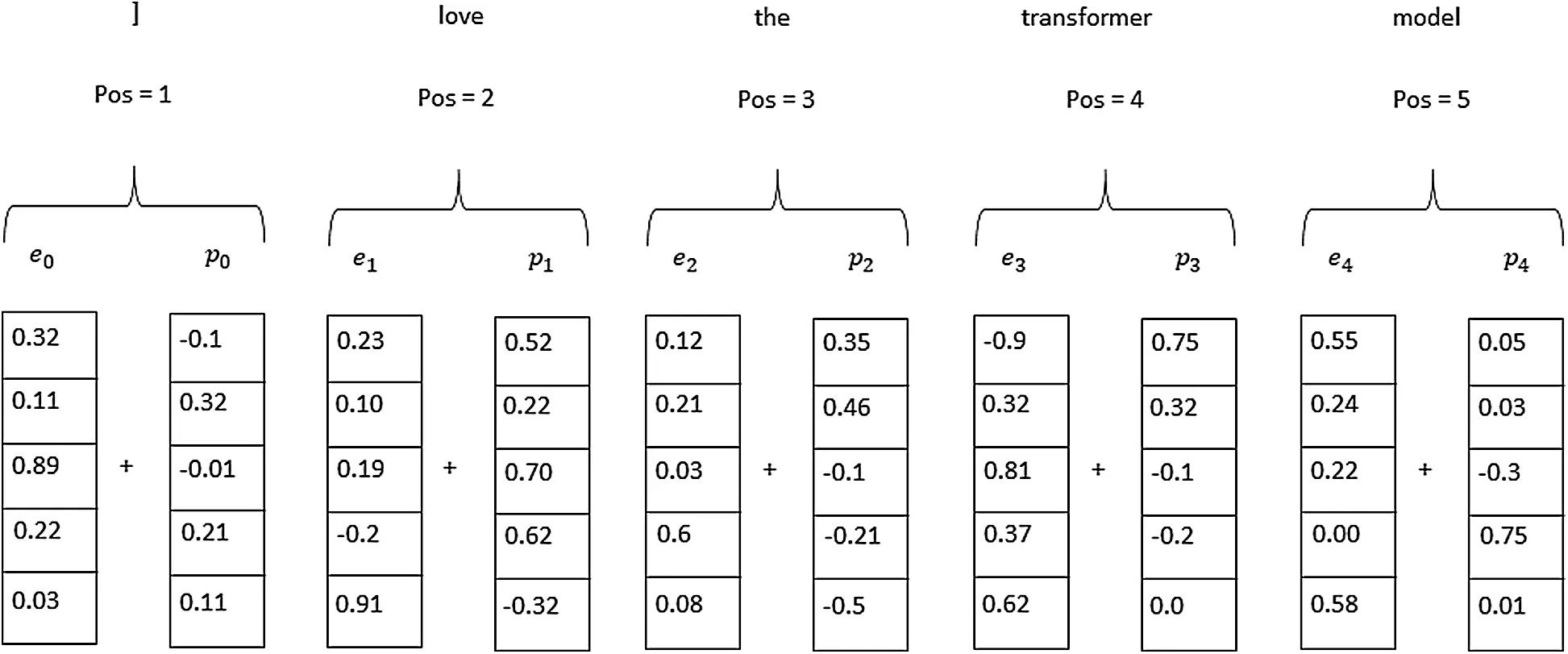Figure 4: Position Encoding steps for “I love the transformer model” sentence

In Multi-Head Attention, the attention function maps a query and a set of key-value pairs for output and all previous values to vectors. The output is computed by calculating a weighted sum of the values. The weight is calculated using the query compatibility function with the corresponding key as shown in Fig. 5. As in , the proposed model adopts the Scaled Dot-Product Attention to calculate the attention function. It has three inputs: queries (Q), keys (K), and values (V). The attention function is defined by Eq. (3).

Attention(Q,K,V)=softmax(QKTdk).V(3)

where Q is queries, K is keys, V is values, and dk is the dimension of keys. The Multi-Head Attention layer enables the model to jointly attend information from different representation subspaces at different positions. This occurs by concatenating heads where a signal head is defined in Eq. (3) and the weight is calculated as Eq. (4).

where headiis a head from a set of heads have size h, WiQ is the weight of headifor queries, WiK is the weight of headi for keys and WiVis the weight of headifor values. The computation of Multi-Head for all heads is followed Eq. (5) where WOis the weight of Multi-Head.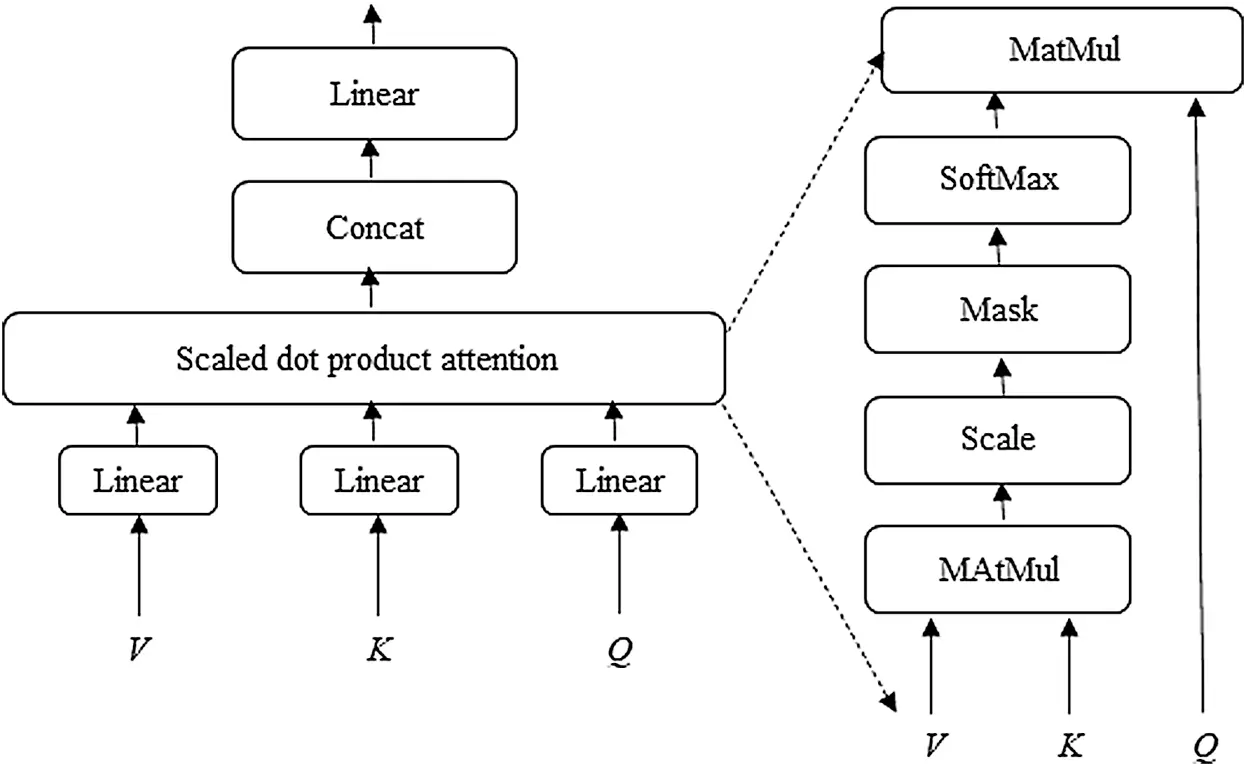Figure 5: Multi-Head Attention consists of several attention layers running in parallel

3.3 Post-Processing

In testing phase, the proposed model corrects Pseudo-code as tokenizer by adding spaces between tokens; such as, (\'\"\ ⋁/?|&<>∗+%). For example, the translation of code “ s = s . replace ( ′\r″ , ′\ \r″ ).” is “ replace every occurrence of ′\r ′ in s with ′\ \ r ′.”, this well be corrected to “ replace every occurrence of ′\r″ in s with ′\ \r″.” as shown in Fig. 6.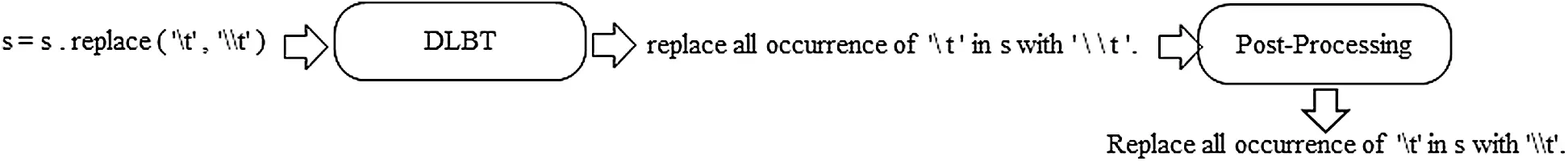Figure 6: Example of Pseudo-code after Post-processing

4  Experimental Results and Evaluation

4.1 Dataset Description

The proposed deep learning-based transformer (DLBT) model is evaluated using Django codebase . It is gathered from a web application framework. The Django dataset contains more than 18,800 lines of a source code written in Python and corresponds to the English Pseudo-code of those lines. A sample of the dataset is shown in Fig. 7. DLBT is evaluated using Method(1) and Method(2) tokenizers as follows:

●   Method(1) tokenizer: the vocabulary sizes of Pseudo-code and Python code are 10,867 and 6,226 respectively. The maximum sequence lengths of Pseudo-code and the source code are 538 and 963 respectively.

●   Method(2) tokenizer: the vocabulary sizes of Pseudo-code and Python code are13,060 and 8,552. The maximum sequence lengths are 522 and 613.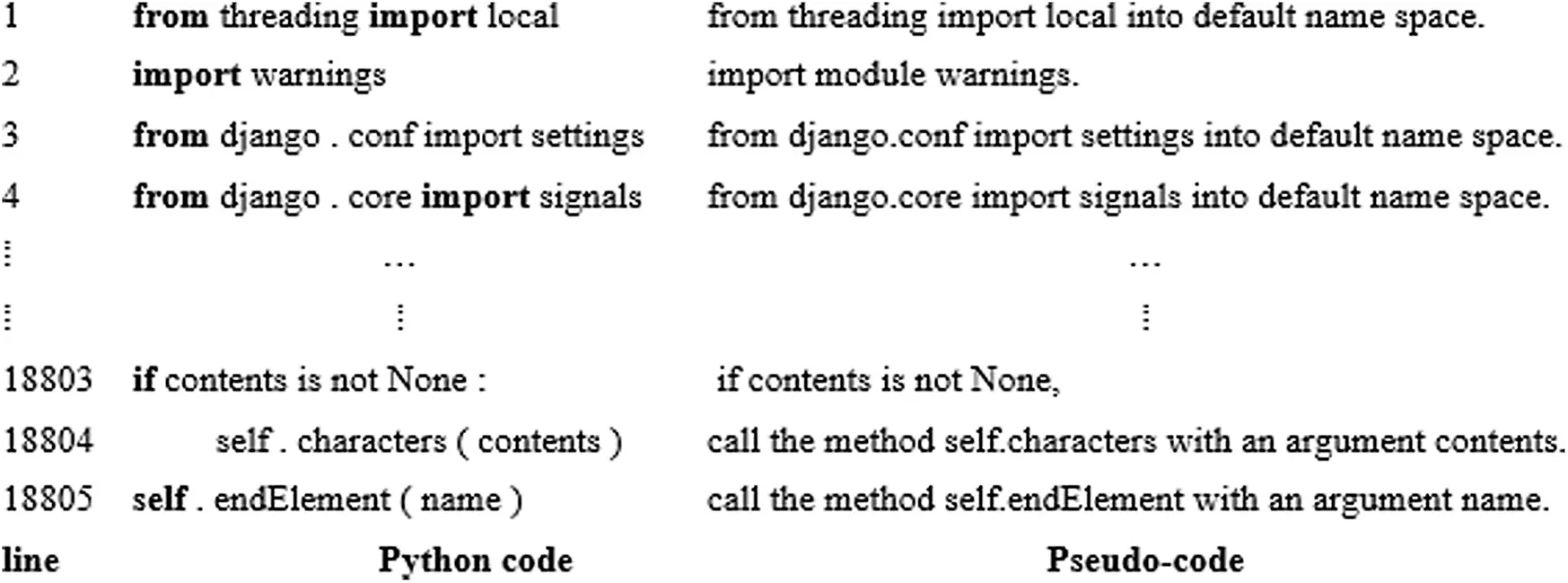Figure 7: Example of Django codebase

The proposed DLBT model is implemented by PyTorch  for deep learning and Spacy  for natural language processing. WordNet  is used to tokenizing the source code lines. DLBT is assessed with two values for the number of layers in the encoder and decoder(6-layers and 8-layers).

4.2 Performance Measures

The BLEU score is a metric measurement to evaluate the quality of machine translation techniques. Quality is measured in terms of how to match between the machine translation output (candidate or hypothesis) and human translation output (reference). BLEU score is a value, ranging from 0 to 1. The machine-translation output closest to the human translation output gets a high BLEU score. If the machine translation output is identical to the human translation output, then the BLEU score will be 1. The BLEU uses a modified form of precision uni-gram, bi-gram, 3-gram, and 4-gram to compare a candidate translation against multiple reference translations.

Pn=ngramϵCcountclip(ngram)ngramϵCcount(ngram)(9)

where Pn is the precision score, ngramϵCcountclip(ngram) is the n-gram matches sentence by sentence. Additionally, the clipped n-gram counts for all the candidate sentences. ngramϵCcountclip(ngram)is the number of candidate n-grams in the test dataset.

BP={e(1|r||c|)if|r||c|1otherwise}(10)

BP is the brevity penalty, |r| is the reference dataset length, |c| is the candidate translation length.

BLEU=BP.exp(n=1Nwn.logPn)(11)

Using n-grams up to length N and positive weights n=1Nwn summing to one. Accuracy is calculated by summing the value of all the sentences equal to 1 in the BLEU score and dividing by the number of sentences.

4.3 Results

Tab. 2 shows an output example of generating Pseudo-code from source code using the DLBT model. Experiments are conducted to show the performance of the proposed model using six-layers and eight-layers for the encoder-decoder structure. Tab. 1 is divided to two columns. Column one contains five lines of Python code. The second column is the Pseudo-code column. Pseudo-code has three forms: the manual output using the professional programmer, the output of DLBT six-layers, and the last one is the output from DLBT eight-layers. Both six-layers and eight-layers generate the correct Pseudo-code for line 1 and line 2 code. Moreover, they avoid the mistake that humans may make; such as the name of package “gzip”, the name of class “GzipFile”, the name of method “__init__”, the number of argument and the name “self” “text”.

In line 3, the six-layer DLBT misses the two-argument “col” and “area”. Eight-layers DLBT prevents this error because it has more training, it is formed of more than six-layers DLBT. In lines 4 and 5, the six layers-DLBT add “s is an empty list” in both lines and some other mistakes. In line 5, The eight-layers DLBT change"every” by “all”. This is a mistake compared with the manually generated Pseudo-code. It is a logic error because DLBT uses WordNet in the tokenization phase and the two words have close meaning and are semantically related.

Moreover, cross-validation is added to the proposed DLBT and is evaluated in terms of accuracy and BLEU performance measures, as shown in Tab. 3 Six-layers DLBT records 36.85, 36.42 accuracy, and 59.62. 59.31 BLEU measures when applying Method(1) and method(2) for tokenization. Using cross-validation and applying Method(1) and Method(2), the DLBT accuracy reaches 46.89 and 49.94 for Method(1) and Method(2), respectively. BLEU reaches 67 and 67.18. In eight layers of DLBT, accuracy and BLEU are reported respectively 35.13, 58.58 for Method(1) and 34.8, 57.92 for Method(2). Using cross-validation and Method(1), it records 47.06, 68.31 accuracy and BLEU measures, respectively. Using cross-validation and Method(2), it records 47.32, 68. 49 accuracy and BLEU measures, respectively. The higher performance of DLBT results when applying cross-validation and Method(2) for tokenization. Fig. 8 presents a comparison for DLBT performance when applying cross-validation and Method(2) tokenization for six-layers and eight-layers.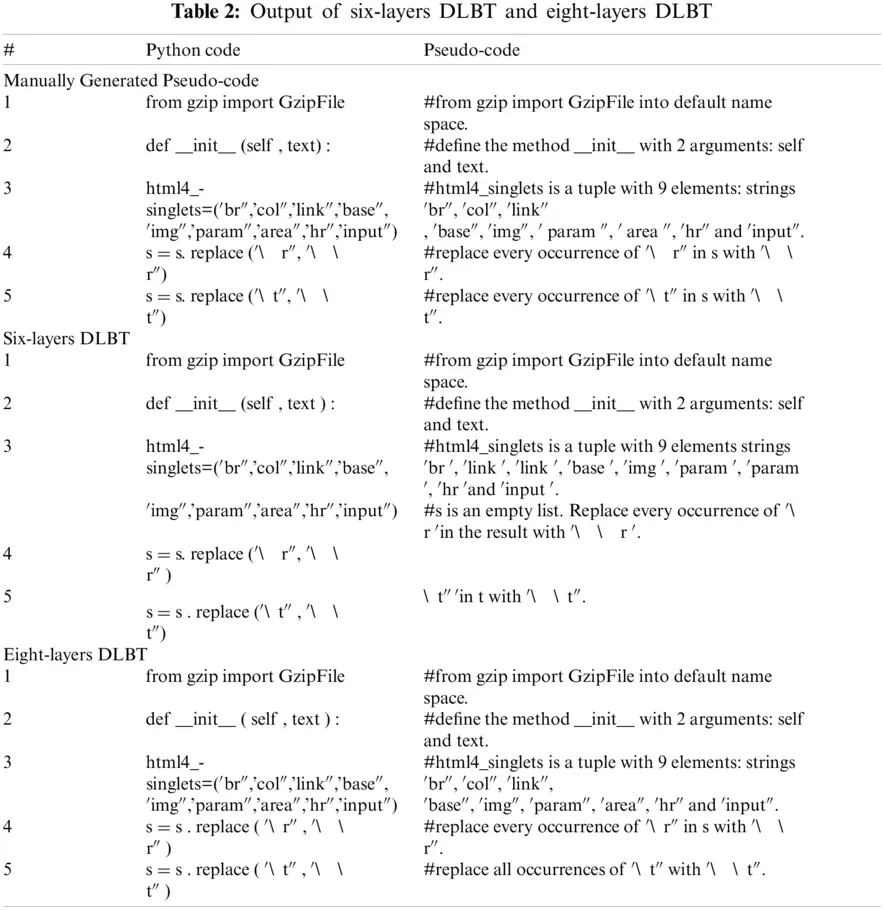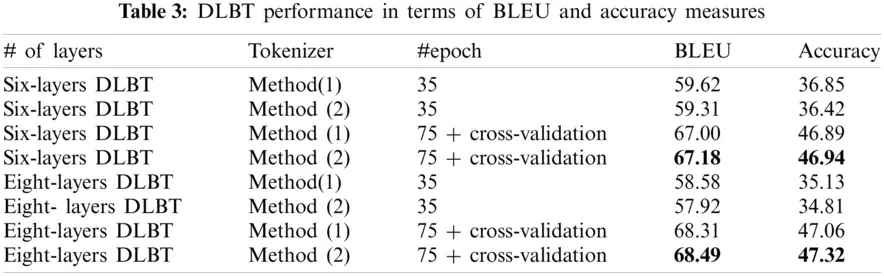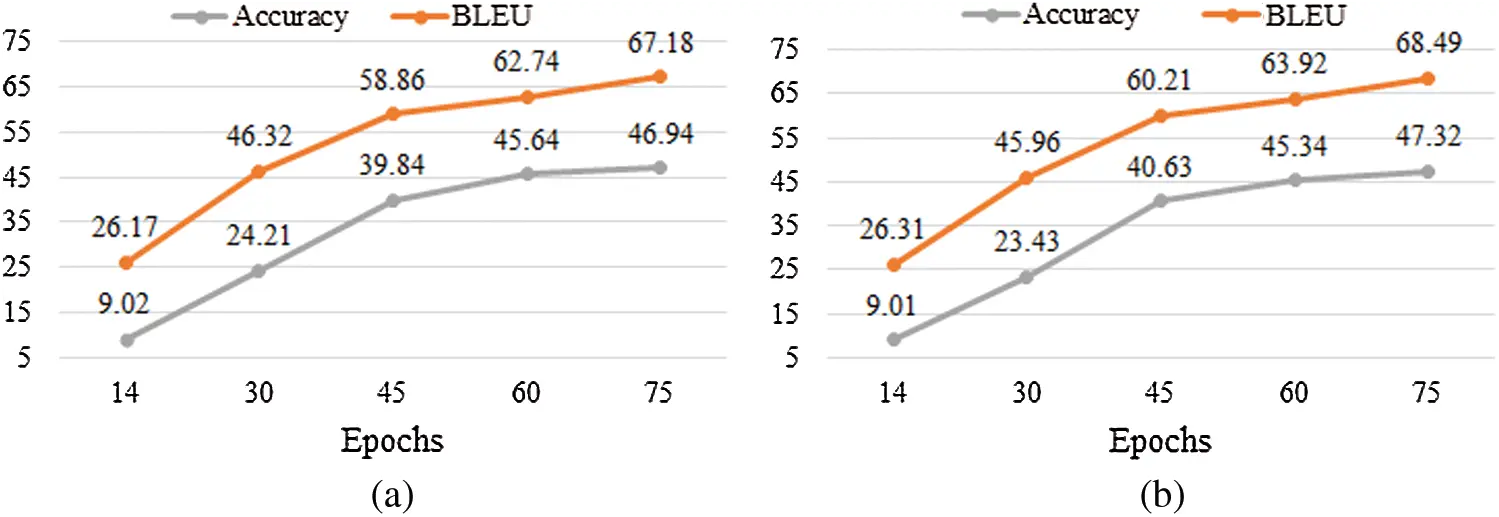Figure 8: DLBT performance using Method(2) and cross validation. (a) Six-layers. (b) Eight-layers

Tab. 4 and Fig. 9 show the results of six-layers DLBT and eight-layers DLBT compared to other methods:code 2 Pseudo-code , Tree-to-String Machine Statistical Machine Translation (T2SMT) , Phrase-Based Machine Translation (PBMT) , Code2NL , Code-GRU, Seq2Seq , and Rule-Based Machine Translation (RBMT) .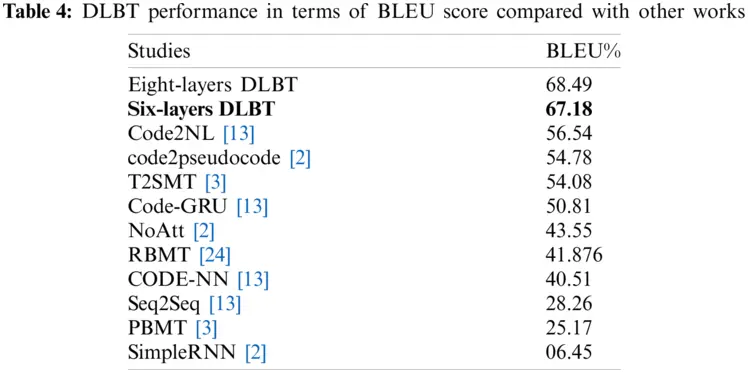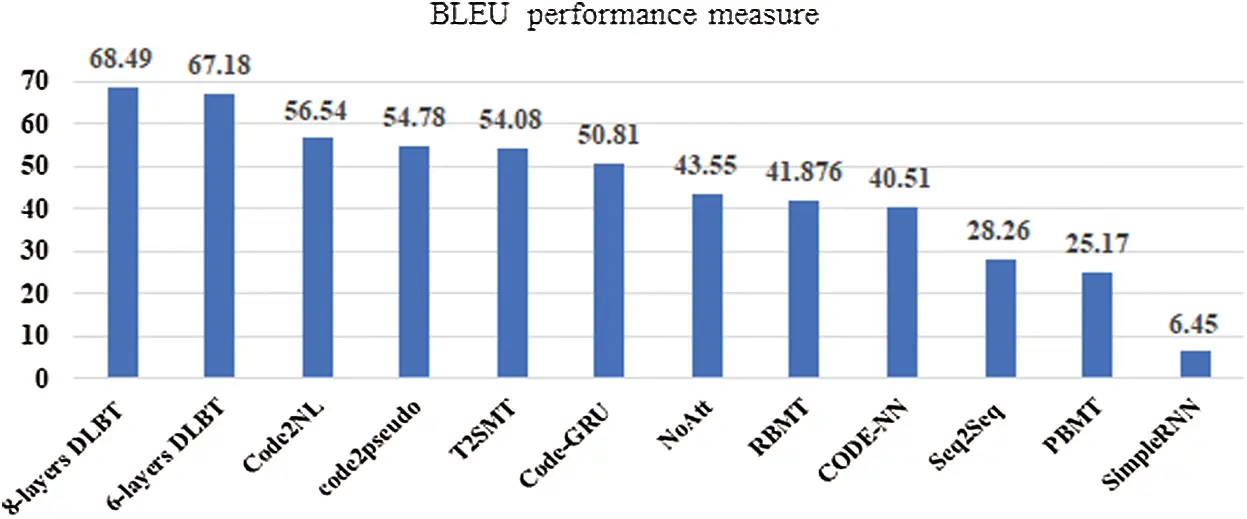Figure 9: DLBT performance in terms of BLEU score compared with other works

4.4 Results Discussion and Interpretation

In , the authors introduced models that are based on Recurrent Neural Network (RNN). The input for the network layers is the output of earlier layers. In training, each sentence is tokenized and assigned to input layers in sequence, one word at a time. This means information moves through time in RNNs, which means that information from earlier time points is used as input for the next subsequent time points. The training for the time point t is happening all along based on inputs that are coming from untrained layers. Thus, because of the vanishing gradient, the whole network is not being trained properly. In , LSTM (long short-term memory) is added to remember the information for a long time to fix the RNN problem. models are considered a sequential network and handle the vanish gradient problem encountered by RNN. In , self-attention is added to improve results and capture the long-term dependencies in source code and Pseudo-code. The RNN-based sequence models have two limitations:

●   They do not model the non-sequential structure of source code as they process the code tokens sequentially.

●   Source code can be very long, and thus these models may fail to capture the long-range dependencies between code tokens.

The proposed DLBT outperforms the performance of the previous models because of

●   Tokenization: the rule expression for Tokenizer is ()

●   Positional embeddings: it is position-insensitive and positional encoding is used to guarantee the order relationship between words in the text;

●   Multi-head attention mechanism: each input is divided into multiple heads, and each head uses the attention mechanism. This unit is used to compute similarity scores between words in a sentence;

●   Cross-validation: it scans the dataset and defines the dataset to test the DLBT in the training phase (validation dataset). It reinforces the vocabulary that is not defined in the training data and put in the test data.

●   Post-processing: it is an important process in the testing phase. It finds the mathematical expressions errors and corrects them such as spaces between a single quotation or double quotation.

5  Conclusions and Future Work

In this paper, a novel deep learning-based transformer (DLBT) model is proposed for automatic Pseudo-code generation from the source code. The proposed DLBT is an encoder-decoder structure, which consists of three components: Tokenization and Embedding, Transformer (encoding and decoding), and Post-processing. In the tokenization and embedding step, the DLBT manages the order and relationship between the tokens. Then, the encoder-decoder structure is built using a multi-head attention mechanism to calculate a score of similarity between the tokens. Finally, the correction function is applied. The proposed model is evaluated using a large dataset of Python code. The proposed model is assessed using six and eight layers and applying cross-validation. The experimental results are promising compared to other methods. Using six and eight layers, it reaches 68.49% and 67.18% in terms of BLEU score performance measures, respectively.

We are planning to add more languages such as C++, C#, and Java. In addition, we want to enhance the proposed DLBT to deal with more complex code rather than simple lines of code and output more abstract description. Moreover, there will be an important step to discover syntax errors before the preprocessing step. This step guarantees that the source code is correct before generating the Pseudo-code. Using this information can partially enhance Pseudo-code generation and help to increase accuracy and BLEU performance measures.

Funding Statement: The authors received no specific funding for this study.

Conflicts of Interest: The authors declare that they have no conflicts of interest to report regarding the present study.

## References

1. N. Al-Saiyd, “Source code comprehension analysis in software maintenance,” in Proc. ICCCS, Krakow, Poland, pp. 1–5, 2017.
2. A. Alhefdhi, H. Dam, H. Hata and A. Ghose, “Generating pseudo-code from source code using deep learning,” in Proc. 25th ASWEC, Adelaide, SA, Australia, pp. 21–25, 2018.
3. Y. Oda, H. Fudaba, G. Neubig, H. Hata, S. Sakti et al., “Learning to generate pseudo-code from source code using statistical machine translation,” in Proc. 30th ACM ASE, Lincoln, NE, USA, pp. 574–584, 2015.
4. T. Iqbal and S. Qureshi, “The survey: Text generation models in deep learning,” Journal of King Saud University—Computer and Information Sciences, 2020.
5. M. Roodschild, J. Sardiñas and A. Will, “A new approach for the vanishing gradient problem on sigmoid activation,” Progress in Artificial Intelligence, vol. 9, pp. 351–360, 2020.
6. S. Hochreiter and J. Schmidhuber, “Long short-term memory,” Neural Computation, vol. 9, no. 8, pp. 1735–1780, 1997.
7. M. Sundermeyer, R. Schlüter and H. Ney, “LSTM neural networks for language modeling,” in Proc. INTERSPEECH, Aachen, Germany, pp. 194–197, 2012.
8. P. Le and W. Zuidema, “Quantifying the vanishing gradient and long-distance dependency problem in recursive neural networks and recursive LSTMs,” in Proc. 1st Workshop on Representation Learning for NLP, Berlin, Germany, pp. 87–93, 2016.
9. A. Vaswani, N. Shazeer, N. Parmar, J. Uszkoreit, L. Jones et al., “Attention is all you need,” in Proc. NIPS, Long Beach, CA, USA, 2017.
10. E. Reiter, “A structured review of the validity of BLEU,” Computational Linguistics, vol. 44, no. 3, pp. 393–401, 2018.
11. D. Bahdanau, K. Cho and Y. Bengio, “Neural machine translation by jointly learning to align and translate,” in Proc. ICLR, San Diego, CA, 2015.
12. L. Büch and A. Andrzejak, “Learning-based recursive aggregation of abstract syntax trees for code clone detection,” in Proc. 26th SANER, Hangzhou, China, pp. 95–104, 2019.
13. Y. Deng, H. Huang, X. Chen, Z. Liu, S. Wu et al., “From code to natural language: Type-aware sketch-based seq2seq learning,” in Proc. DASFAA, Jeju, South Korea, pp. 352–368, 2020.
14. J. Gu, Z. Lu, H. Li and V. Li, “Incorporating copying mechanism in sequence-to-sequence learning,” in Proc. the 54th Annual Meeting of the Association for Computational Linguistics, Berlin, Germany, vol.1, pp. 1631–1640, 2016.
15. P. Yin and G. Neubig, “A syntactic neural model for general-purpose code generation,” in Proc. The 55th ACL, Vancouver, Canada, pp. 440–450, 2017.
16. S. Hayati, R. Olivier, P. Avvaru, P. Yin, A. Tomasic el al., “Retrieval-based neural code generation,” in Proc. Empirical Methods in Natural Language Processing, Brussels, Belgium, pp. 925–930, 2018.
17. J. Zhang, M. Utiyama, E. Sumita, G. Neubig and S. Nakamura, “Guiding neural machine translation with retrieved translation pieces,” in Proc. NAACL, New Orleans, Louisiana, vol. 1, pp. 1325–1335, 2018.
18. L. Mou, G. Li, L. Zhang, T. Wang and Z. Jin, “Convolutional neural networks over tree structures for programming language processing,” in Proc. AAAI, Arizona, USA, vol. 2, no. 3, pp. 1287–1293, 2016.
19. X. Hu, G. Li, X. Xia, D. Lo and Z. Jin, “Deep code comment generation,” in Proc. 40th ICSE, New York, NY, United States, pp. 200–210, 2018.
20. X. Hu, G. Li, X. Xia, D. Lo and Z. Jin, “Deep code comment generation with hybrid lexical and syntactical,” Empirical Software Engineering, vol 25, pp. 2179–2217, 20
21. X. Yu, Q. Huang, Z. Wang, Y. Feng and D. Zhao, “Towards context-aware code comment generation,” Association for Computational Linguistics: EMNLP, pp. 3938–3947, 2020.
22. K. Cho, B. Merriënboer, C. Gulcehre, D. Bahdanau, F. Bougares et al., “Learning phrase representations using RNN encoder-decoder for statistical machine translation,” in Proc. EMNLP, Doha, Qatar, pp. 1724–1734, 2014.
23. P. Velickovic, G. Cucurull, A. Casanova, A. Romero, P. Lio et al., “Graph attention networks,” in Proc. 6th ICLR, Vancouver, BC, Canada, 2018.
24. S. Rai and A. Gupta, “Generation of Pseudo code from the Python source code using rule-based machine translation,” arXiv e-prints, 2019.
25. A. Paszke, S. Gross, F. Massa, A. Lerer, J. Bradbury et al., “Pytorch: An imperative style, high-performance deep learning library,” in Proc. 33rd NeurIPS, Vancouver, Canada, pp. 8024–8035, 2019.
26. SpaCy, “Industrial-Strength Natural Language Processing,” [Online]. Available: https://spacy.io.
27. Princeton University, “WordNet, A Lexical Database,” 2021. [Online]. Available: https://wordnet.princeton.edu.This work is licensed under a Creative Commons Attribution 4.0 International License, which permits unrestricted use, distribution, and reproduction in any medium, provided the original work is properly cited.